# Equations Math problems

#### Number of problems found: 1811

• AlexandraAlexandra made a rectangular quilt the measured 3 1/4. 2 3/4 feet in width. What is the area of the quilt in square feet? Write an equation to solve.
• Three 105Three consecutive integers add up to 51. what are these integers?
• Original numberJoe divided a number by 2 1/4 to get 2/3. What was the original number?
• The product 2The product of two functions is 10. If one of them is 2 1/3, find the other one.
• Fraction equationEx over four is equal to two and one-fifth times ex plus three over ten. What is ex>
• Frac equation v23.80 is two fifths of a quarter sum of money. How much money is there?
• Two fraction equation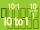Find d value of q in the equation 30/16=q/48.
• A class IV.CIn a class 2/5 were boys. 30 were girls. How many more girls than boys are there?
• Supplementary angles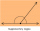(a + 30)° and (2a)° are the measure of two supplementary angles. Find the value of 'a'.
• Six times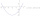6 times a number has 2 subtracted from it. The result it thrilled . the answer is the same if you add 6 to twice the number and multiply the result by 2
• Over fractionSolve equation: p over 5 equals fraction numerator 7 p over denominator 6 end fraction plus 5
• Regular price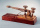The sale price of a television set is 7200. The discount rate is 40%. Find its regular price.
• Taken away from number18 is taken away from 8 times of a number is 30. Find the number.
• The sum 14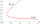The sum of five times a number and 10 is equal to the difference of the number and 10.
• Conjugate equation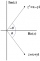Find the numbers of a and b, if (a - bi) (3 + 5i) is the Conjugate of (-6 - 24i)
• Points OPQ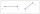Point P is on line segment OQ. Given OP = 6, OQ = 4x - 3, and PQ = 3x, find the numerical length of OQ.
• SixteenSixteen minus the quotient of 12 and what number is thirteen?
• Fraction eq2/3x + 5/7 = 1/2x + 22/21
• The ratio 4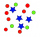The ratio of two number is 5:4 if 40% of the first number is 12, what will be 50% of the second number?
• Eq2 equationsFor each of the following problems, determine the roots of the equation. Given the roots, sketch the graph and explain how your sketch matches the roots given and the form of the equation: g(x)=36x2-12x+5 h(x)=x2-4x+20 f(x)=4x2-24x+45 p(x)=9x2-36x+40

Do you have an exciting math question or word problem that you can't solve? Ask a question or post a math problem, and we can try to solve it.

We will send a solution to your e-mail address. Solved examples are also published here. Please enter the e-mail correctly and check whether you don't have a full mailbox.

Please do not submit problems from current active competitions such as Mathematical Olympiad, correspondence seminars etc...

Do you have a linear equation or system of equations and looking for its solution? Or do you have a quadratic equation?